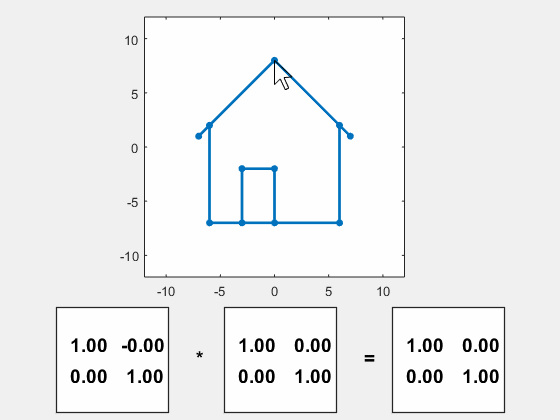# Matrix Multiplication Flexes House

A new app employs transformations of a graphic depicting a house to demonstrate matrix multiplication.

### Contents#### Strang's House

The house in the animated gif has been featured in Gil Strang's textbook, Introduction to Linear Algebra. A quilt inspired by the house in on the cover of the third edition.

#### Rotate and stretch

You can rotate the house with your mouse by clicking and dragging the peak of the roof. You can stretch the house horizontally by clicking and dragging either side. You can stretch the house vertically by dragging the floor.

#### Matrix multiplication

The motion is effected by matrix multiplication. Rotating the roof through an angle $\theta$ defines a 2-by-2 orthogonal matrix $U$.

$$U = \left( \begin{array}{rr} \cos\theta \ \ \sin\theta \\ -\sin\theta \ \ \cos\theta \\ \end{array} \right)$$

Moving a side defines a horizontal scaling $\sigma_1$. Moving the floor defines a vertical scaling $\sigma_2$. Together they form the diagonal scaling matrix $S$.

$$S = \left( \begin{array}{rr} \sigma_1 \ \ 0 \\ 0 \ \ \sigma_2 \\ \end{array} \right)$$

The resulting 2-by-2 transformation matrix $A$ is the product of $U$ and $S$.

$$A = U S$$

Watch how the three matrices change as you manipulate the house. The scale factor $\sigma_1$ operates on the first column of $U$, while $\sigma_2$ scales the second.

#### Code

The code for this app is available here.

Published with MATLAB® R2016a

|